### Learning Outcomes

• Graph quadratic functions using tables and transformations
• Identify important features of the graphs of quadratic functions

Quadratic functions can also be graphed. It is helpful to have an idea about what the shape should be so you can be sure that you have chosen enough points to plot as a guide. Let us start with the most basic quadratic function, $f(x)=x^{2}$.

## Graphing Quadratic Functions Using Tables

Graph $f(x)=x^{2}$.
Start with a table of values. Then think of each row of the table as an ordered pair.

x f(x)
$−2$ $4$
$−1$ $1$
$0$ $0$
$1$ $1$
$2$ $4$

Plot the points $(-2,4), (-1,1), (0,0), (1,1), (2,4)$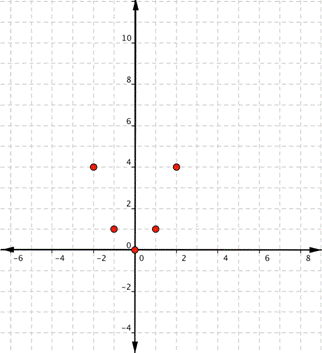Since the points are not on a line, you cannot use a straight edge. Connect the points as best as you can using a smooth curve (not a series of straight lines). You may want to find and plot additional points (such as the ones in blue here). Placing arrows on the tips of the lines implies that they continue in that direction forever.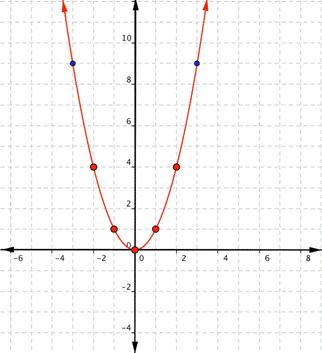The equations for quadratic functions have the form $f(x)=ax^{2}+bx+c$ where $a\ne 0$. In the basic graph above, $a=1$, $b=0$, and $c=0$.

In the following video, we show an example of plotting a quadratic function using a table of values.

## Graphing Quadratic Functions Using Transformations

Notice that the shape of $f(x)=x^2$ is similar to the letter U. This is called a parabola. One-half of the parabola is a mirror image of the other half. The lowest point on this graph is called the vertex. The vertical line that goes through the vertex is called the line of reflection. In the case of $f(x)=x^2$, that line is the y-axis.  All quadratic functions have graphs in the shape of a parabola.  However, the values of $a$, $b$, and $c$ change the direction, shape, and position of the graph.  below, we will discuss how changing these values can transform a graph.

### How $a$ affects the graph of $f(x)=ax^{2}+bx+c$

The value of $a$ tells us whether the parabola opens upwards ($a>0$) or downwards ($a<0$). If $a$ is positive, the vertex is the lowest point on the graph and the graph opens upward.  If a is negative, the vertex is the highest point on the graph and the graph opens downward.

The value of $a$ also tells us about the width the graph.  When $|a|>1$ the graph will appear more narrow than $f(x)=x^2$.  When $|a|<1$ the graph will appear wider than  $f(x)=x^2$.  In the following example, we show how changing the value of $a$ will affect the graph of the function.

### Example

Match each function with its graph.

a) $\displaystyle f(x)=3{{x}^{2}}$

b) $\displaystyle f(x)=-3{{x}^{2}}$

c) $\displaystyle f(x)=\frac{1}{2}{{x}^{2}}$

1)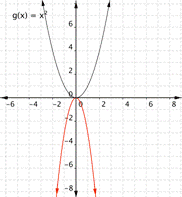2)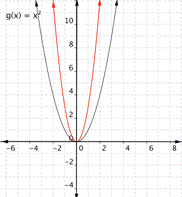3)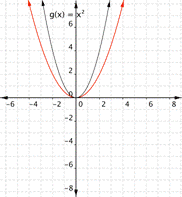### How $b$ affects graphs of quadratic functions

When a quadratic function is in the form $f(x)=ax^2+c$, meaning there is no b term, changing c moves the parabola up or down along the y-axis. In the next example, we show how changes to affect the graph of the function.

### Example

Match each of the following functions with its graph.

a) $\displaystyle f(x)={{x}^{2}}+3$

b) $\displaystyle f(x)={{x}^{2}}-3$

1)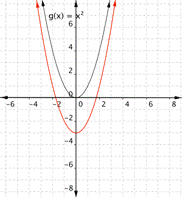2)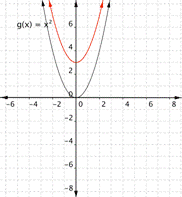When we are graphing any quadratic function, it is also useful to know that $c$ corresponds to the y-intercept of any quadratic function.  The y-intercept occurs when $x=0$, or at the point $(0,f(0)$.  Recall that we determine the value of $f(0)$ by plugging $0$ into our equation:

$f(0)=a(0)^2+b(0)+c=c$

For a quadratic equation, the y-intercept is the point $(0,c)$.

### Finding the Vertex of a Parabola

Changing $b$ moves the line of reflection, which is the vertical line that passes through the vertex (the high or low point) of the parabola. It may help to know how to calculate the vertex of a parabola to understand how changing the value of $b$ in a function will change its graph.

To find the vertex of the parabola, use the formula $\displaystyle \left( \frac{-b}{2a},f\left( \frac{-b}{2a} \right) \right)$.

For example, if the function being considered is $f(x)=2x^2-3x+4$, to find the vertex, first calculate $\dfrac{-b}{2a}$

$a = 2$, and $b = -3$, therefore $\dfrac{-b}{2a}=\dfrac{-(-3)}{2(2)}=\dfrac{3}{4}$.

This is the $x$ value of the vertex.

Now evaluate the function at $x =\dfrac{3}{4}$ to get the corresponding y-value for the vertex.

$f\left( \dfrac{-b}{2a} \right)=2\left(\dfrac{3}{4}\right)^2-3\left(\dfrac{3}{4}\right)+4=2\left(\dfrac{9}{16}\right)-\dfrac{9}{4}+4=\dfrac{18}{16}-\dfrac{9}{4}+4=\dfrac{9}{8}-\dfrac{9}{4}+4=\dfrac{9}{8}-\dfrac{18}{8}+\dfrac{32}{8}=\dfrac{23}{8}$.

The vertex is at the point $\left(\dfrac{3}{4},\dfrac{23}{8}\right)$.  This means that the vertical line of reflection passes through this point as well.  It is not easy to tell how changing the values for $b$ will change the graph of a quadratic function, but if you find the vertex, you can tell how the graph will change.

In the next example, we show how changing can change the graph of the quadratic function.

### Example

Match each of the following functions with its graph.

a) $\displaystyle f(x)={{x}^{2}}+2x$

b) $\displaystyle f(x)={{x}^{2}}-2x$

a)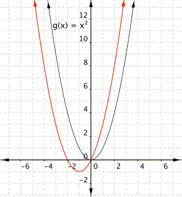b)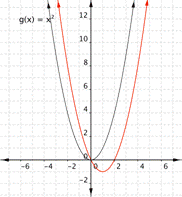Note that the x-value of the vertex is not dependent upon the value of $c$ in a quadratic function.  However, the vertex does change if the value for $c$ changes because the y-value of the vertex is calculated by substituting the x-value into the function. Here is a summary of how the changes to the values for a, b, and c of a quadratic function can change it is graph.

## Features of a Parabola

We have learned some important things about the graphs of quadratic functions that will make it easier for us to create a graph of a quadratic function.  These features of a parabola are summarized in the textbox below:

### Important Features of the Graphs of Quadratic Functions

For $\displaystyle f(x)=a{{x}^{2}}+bx+c$, where a, b, and c are real numbers,

• The parabola opens upward if $a > 0$ and downward if $a < 0$.
• a changes the width of the parabola. The parabola gets narrower if $|a|> 1$ and wider if $|a|<1$.
• The y-intercept of the parabola occurs at the point $(0,c)$.
• The vertex depends on the values of a, b, and c. The vertex is $\left(\dfrac{-b}{2a},f\left( \dfrac{-b}{2a}\right)\right)$.

In the last example, we showed how you can use the properties of a parabola to help you make a graph without having to calculate an exhaustive table of values.

### Example

Graph $f(x)=−2x^{2}+3x–3$.

The following video shows another example of plotting a quadratic function using the vertex.

## Summary

Creating a graph of a function is one way to understand the relationship between the inputs and outputs of that function. Creating a graph can be done by choosing values for x, finding the corresponding y values, and plotting them. However, it helps to understand the basic shape of the function. Knowing how changes to the basic function equation affect the graph is also helpful.

The shape of a quadratic function is a parabola. Parabolas have the equation $f(x)=ax^{2}+bx+c$, where a, b, and c are real numbers and $a\ne0$. The value of a determines the width and the direction of the parabola, while the vertex depends on the values of a, b, and c. The vertex is $\displaystyle \left( \dfrac{-b}{2a},f\left( \dfrac{-b}{2a} \right) \right)$.

## Contribute!

Did you have an idea for improving this content? We’d love your input.